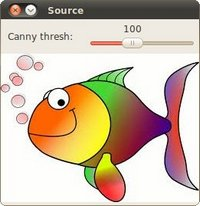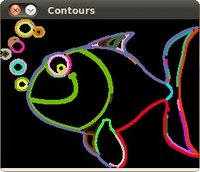OpenCV  3.4.0 Open Source Computer Vision

## Goal

In this tutorial you will learn how to:

## Code

This tutorial code's is shown lines below. You can also download it from here

#include <iostream>
using namespace cv;
using namespace std;
Mat src; Mat src_gray;
int thresh = 100;
int max_thresh = 255;
RNG rng(12345);
void thresh_callback(int, void* );
int main( int argc, char** argv )
{
String imageName("../data/happyfish.jpg"); // by default
if (argc > 1)
{
imageName = argv;
}
if (src.empty())
{
cerr << "No image supplied ..." << endl;
return -1;
}
cvtColor( src, src_gray, COLOR_BGR2GRAY );
blur( src_gray, src_gray, Size(3,3) );
const char* source_window = "Source";
namedWindow( source_window, WINDOW_AUTOSIZE );
imshow( source_window, src );
createTrackbar( " Canny thresh:", "Source", &thresh, max_thresh, thresh_callback );
thresh_callback( 0, 0 );
waitKey(0);
return(0);
}
void thresh_callback(int, void* )
{
Mat canny_output;
vector<vector<Point> > contours;
vector<Vec4i> hierarchy;
Canny( src_gray, canny_output, thresh, thresh*2, 3 );
findContours( canny_output, contours, hierarchy, RETR_TREE, CHAIN_APPROX_SIMPLE, Point(0, 0) );
Mat drawing = Mat::zeros( canny_output.size(), CV_8UC3 );
for( size_t i = 0; i< contours.size(); i++ )
{
Scalar color = Scalar( rng.uniform(0, 255), rng.uniform(0,255), rng.uniform(0,255) );
drawContours( drawing, contours, (int)i, color, 2, 8, hierarchy, 0, Point() );
}
namedWindow( "Contours", WINDOW_AUTOSIZE );
imshow( "Contours", drawing );
}

## Result

Here it is: